# Analysis the Impact of High Frequency Harmonics in Power Line Communication

9177 words (37 pages) Essay in Engineering

18/05/20 Engineering Reference this

Disclaimer: This work has been submitted by a student. This is not an example of the work produced by our Essay Writing Service. You can view samples of our professional work here.

Any opinions, findings, conclusions or recommendations expressed in this material are those of the authors and do not necessarily reflect the views of UK Essays.

Analysis the Impact of High frequency Harmonics in Power Line Communication

## Abstract

Power Line Communication (PLC) is a technology where transmission of data and voice signals along with electrical signals in new or existing power lines. PLC was analysed and its mathematical modelling was determined in the project. In this project the power line that was used for analysis is a two-wire transmission line through which behavior of signals transmitted on PLC channel were analysed. The aim of the project is to analyse the effect of harmonics in the power line communication. By modelling the power line communication with time domain and frequency domain modelling, the signal characteristics of the power line communication has been investigated. The mathematical modelling approaches applied were multipath and two-wire transmission line. Then, adding harmonics to those models can identify how the signals get affected and the power line communication signals getting distorted by harmonics injected has been investigated. Simulink tool in MATLAB software was used for simulations and graphs plots of magnitude response and phase response presented and compared with the theoretical plots and graphs. It is determined that the theoretical and simulated phase and magnitude responses are similar and therefore to conclude that it is possible to model access power line networks for PLC implementations.

## Introduction

### Power Line Communication Overview

Power Line Communication (PLC) is a technology where transmission of data and voice signals through existing electrical power distribution lines from one communication system to another. There are two types of PLC communications and include Narrowband PLC and Broadband PLC. The broadband PLC operates for higher frequencies range as 1MHz to about 50MHz and here higher data rates apply like for more than 2Mbps.  A PLC channel transmission system has to convert information data stream in a suitable form before this is injected in the communication channel. A PLC medium introduces attenuation and phase shift on signals, and a PLC medium was designed only for energy distribution, and for this factor several types of machines and appliances are connected to it  .

If you need assistance with writing your essay, our professional essay writing service is here to help!

## Background and Literature Review

Power line communication is normally referred to as transmission of data signals. The low voltage power lines are used as primary transmission lines to transmit multimedia signals, speed data and voice signals. A typical low voltage power line for single phase distribution consists of three conducting cores; phase, neutral and ground. In this project the two-wire transmission line approximates two conductors .

PLC is used to transmit by introduction of signals into the household wiring and the electrical lines. The PLC can be applied in various areas such as computer networks and smart meters etc. The PLC systems operate at a wide range of frequencies such as ultra-narrow band frequency (<3 kHz), narrow band frequency (3 kHz to over 500 kHz) and Broadband frequency (1MHz to 50MHz)  . Broadband powerline communications technologies fall into two main categories; access and in-house power line network. Access power line technologies are used for sending data over low voltage system. And, In-house powerline communication technology transfers data exclusively within the building and extends to all socket outlets within the house . Broadband powerline communication systems can give in-house interconnection of computers and peripherals, and cost-effective long-distance delivery of broadband data services. The data transfer bound on power lines is due to the fact that existing power signals which create Electromagnetic Interference (EMI) with communication signals, and secondly, non-linearity of the power line itself. Furthermore, because of its time-dispersive nature it creates frequency selective fading effect which may give rise to Inter-Symbol Interference (ISI) . The speed of data transfer and frequencies used to transfer the data across the electrical supply network are used to make this type of PLC different from the previous systems used in PLC which is 10 – 40 times slower than this broadband powerline communication. Figure below show the structure of broadband powerline communication network. The broadband powerline communication channel suffers from attention which is frequency dependent and it is produced by line conductor and dielectric losses. The applications of this communication are divided into two categories, in-house applications and last-mile application. In the house applications both the receiver and transmitter in the same build whereas in the last-mile application the PLC transfers data over a long distance .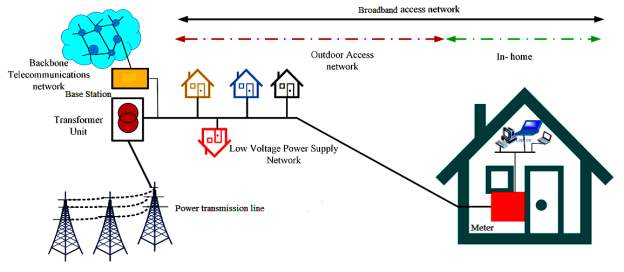Electrical Power Lines were designed for transmission of power having a very low frequency at 50Hz or 60Hz, while broadband signal has a high frequency, but a broadband PLC system has its own limits such as it deals a challenging environment for communication. The noise, line impedances and attenuation vary with time, frequency and location thus making it difficult to model broadband PLC channels .

Power-line carrier (PLC) systems have proven to be susceptible to interference caused by harmonics produced by loads, such as HVDC converters, dc electric arc furnaces, static var compensators, and motor speed controllers. The harmonics are generated by the switching operation of large power converters, such as Static Compensator (STATCOM), Static Reactive Power Controller (SVC) and UPFC. These harmonics may cover a wide range of frequencies and it can cause problems of interference with communication systems. Power Line Carrier (PLC) system is one of the systems used in transmission of signals for Tele-potations, Tele-tripping, Tele-control and speech communications. The Signal to Noise Ratio (SNR) of the PLC is based on the noise level at the input of the carrier receiver   . These effects of harmonics result in overheating, extra losses in electric machines and capacitors; and over voltage due to excited resonance in the power system. These harmonics can be propagated throughout the entire interconnected power network.

To study impacts of harmonics on a PLC, power lines and its distribution systems used as transmission channels is factored in. Power lines provide harsh environment for high frequency communication signals, multipath effects produced due to impedance mismatch on PLC channel. Different noise types in a PLC channel such as impulse noise, background noise, narrowband noise, and attenuation of transmitted signals make it necessary to model PLC channel   . There are two approaches via which a PLC channel can be modelled i.e. multipath model and transmission line approach . Signal propagation along power lines does not take a single path from the transmitter to the receiver. Reflections from load points lead to reception of multiple delayed versions (echoes) of transmitted signal. Existing models for the transfer function of power lines are based on two fundamental approaches; frequency domain and time domain . From  deterministic and probabilistic approach is used for harmonic analysis and here, harmonic analysis has been done for both time domain and frequency domain. The frequency domain analysis was used to determine harmonic voltage distortion magnitudes due to line outages while time domain analysis was used in deriving harmonic modelling of power line distribution system.

In this project, two-wire transmission line theory was adopted. Figure 2 shows approximately two-wire transmission line as tapped-delay line filter. In , frequency domain model analysis normally two conductor transmission line models are used. The two-wire transmission line cannot be analysed using Ohm’s Law because current and voltage are varying continuously. An equivalent circuit can be formed, and it will help to get voltage and current relationship as in figure 2 (a).

## Aims and Objectives

Power Line Communications (PLC) is gaining high technological advancement from communication industry and electric power supply agencies and companies due to demand response, demand side management and advanced metering infrastructure (AMI).

The main aim of this project is to do PLC channel modelling for utilizing power lines and carry out analysis of the impacts of high frequency harmonics in the Power Lines Communications (PLC).

Objectives of the Project include

1)     Perform mathematical modelling of PLC and plot calculated results for transfer function and phase response

2)     To implement the PLC model in MATLAB Simulink, inject harmonics and perform simulations for the PLC model

3)     Analysis of the transfer function of PLC channel and impacts of the high frequency harmonics in the PLC modelled in MATLAB Simulink and compare results of the MATLAB simulations with the calculated plots and results

## Design and Research Challenges

The research challenges faced in simulation of the project include;

      Setting up the harmonic generator and controlling them to implement it and be able to analyse the impacts

      Determination or ratio of coupling transformer at signal sending and receiving end

      Displaying the magnitude and phase responses of the calculated plots and simulated plots in one graph paper for easy comparison and definitions

      Difficulty is representing the simulation model with a code and be able to get similar results

## Project Methodology

The two approaches of modelling PLC channel are multipath modelling through which transmitted signals can be analysed . Multipath approach is based on reflections of signals from different branches because of impedance mismatching   . The multipath nature of powerlines arises from presence of several branches and impedance mismatch causing reflections of signals. Also, time domain approach as all paths from which reflections have occurred are considered and these paths are complex, so the approach becomes complicated. For simplicity, low number of paths from the transmission line has been selected to model the PLC channel. By multipath transmission line transmission modelling, the behavior of signals on power line communication channel can be analysed. In this research project, a two-wire transmission line theory approach was adopted. Since the mathematical modelling is not enough to understand the behavior of signals completely therefore graph plots are necessary. Hence some simulations were performed at various frequencies. According to mathematical modelling phase and magnitude response of the signals will be simulated at different frequencies.

## Fundamental concepts, principles and theories

A two- wire transmission line approach of modelling a PLC is used in this project.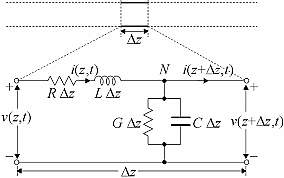Applying Kirchhoff’s Voltage Law and Kirchhoff’s Current Law equations, the values of voltage and current are calculated at z and z+ Δz.

……………………………………….1

………………………………2

Mathematically, as

, above equations become partial derivatives with respect to z giving;

………………………………………………………………3

………………………………………………………………4

For the time harmonic signals, instantaneous voltage and current may be defined in terms of phasor such that

$v\left(z,t\right)=\mathit{Re}\left\{V\left(Z\right){e}^{\mathit{jwt}}\right\}$

……………………………………………………………………5

$i\left(z,t\right)=\mathit{Re}\left\{I\left(Z\right){e}^{\mathit{jwt}}\right\}$

……………………………………………………………………..6

And derivatives of voltage and current with respect to time yields

$j\omega$

times respective phasor which gives

$\frac{\mathit{dV}\left(Z\right)}{\mathit{dZ}}=–\left[R+\mathit{j\omega L}\right]I\left(Z\right)$

……………………………………………………………………7

$\frac{\mathit{dI}\left(Z\right)}{\mathit{dZ}}=–\left[G+\mathit{j\omega C}\right]V\left(Z\right)$

……………………………………………………………………8

Differentiating equation (vii) and (viii) so get current and voltage equations as follows

$\frac{{d}^{2}V\left(Z\right)}{d{Z}^{2}}=–{\gamma }^{2}V\left(Z\right)$

………………………………………………………………………….9

$\frac{{d}^{2}I\left(Z\right)}{d{Z}^{2}}=–{\gamma }^{2}I\left(Z\right)$

…………………………………………………………………………..10

Equations (9) & (10) describe voltage and current on transmission line with respect to distance and time. Where

$\gamma$

is propagation constant of travelling wave on transmission line. Thus propagation constant of two-wire transmission line has been derived and is given below

$\gamma =\alpha +\mathit{j\beta }=\sqrt{\left(R+\mathit{j\omega L}\right)\left(G+\mathit{j\omega C}\right)}$

……………………………………………………..11

The real part

$\alpha$

is attenuation constant and imaginary part

$\beta$

is phase constant. Attenuation constant is defined as the rate at which amplitude of travelling wave is attenuated whereas phase constant is defined rate at which phase of travelling signal is changing.

The general solutions to voltage and current of equations (9) and (10) are given as

$v\left(Z\right)={V}_{0}^{+}{e}^{–\mathit{\gamma z}}+{V}_{0}^{–}{e}^{\mathit{\gamma z}}$

…………………………………………………………………..12

$I\left(Z\right)={I}_{0}^{+}{e}^{–\mathit{\gamma z}}+{I}_{0}^{–}{e}^{\mathit{\gamma z}}$

…………………………………………………………………….13

In equations (12) and (13) superscript plus and minus indicate the travelling wave in +z and –z directions. Relating general solutions with equations (12) and (13) to get

$\frac{{V}_{0}^{+}}{{I}_{0}^{+}}=\frac{{V}_{0}^{–}}{{I}_{0}^{–}}=\frac{R+\mathit{j\omega L}}{\gamma }$

…………………………………………………………………………….14

Replacing

$\gamma$

to get characteristic impedance

$\left({z}_{0}\right)$

can be defined as ratio of voltage to current of a travelling wave on transmission line; it is in form of conductance, resistance, inductance and capacitance.

${z}_{0}=\sqrt{\frac{R+\mathit{j\omega L}}{G+\mathit{j\omega C}}}$

The resistance, conductance, inductance and capacitance values are determined as follows

$R=\frac{1}{\pi \left(\sqrt{\frac{\mathit{\pi f\mu }}{\sigma }}\right)}$

………………………………………………………………………………….15

$G=\frac{\mathit{\pi \sigma }}{\left({\mathit{cos}}^{–1}\left(\frac{D}{2a}\right)\right)}$

………………………………………………………………………………16

$L=\frac{\mu }{\pi \left({\mathit{cos}}^{–1}\left(\frac{D}{2a}\right)\right)}$

……………………………………………………………………………..17

$C=\frac{\mathit{\pi \epsilon }}{\left({\mathit{cos}}^{–1}\left(\frac{D}{2a}\right)\right)}$

………………………………………………………………………………18

For the case when there is more than one path in the transmission line network, reflection factor must be considered of impedance mismatch of various paths

$r=\frac{{Z}_{l}–{Z}_{0}}{{Z}_{l}+{Z}_{0}}$

……………………………………………………………………………………………….19

Where

$r$

is the reflection factor,

${Z}_{l}$

${Z}_{0}$

is characteristic impedance.

From , For a power line transmission, the propagation of data signals follows a multipath following a pattern very similar to wireless signals in a cellular transmission. Considering a Power grid (LV) is a single central transmission line with shooting stems terminating at the end user place, and a small section for a multipath propagation of signal in different routes, the signal power and Bit Error Rate(BER) of received signal depends upon the path followed and length of path. Multipath propagation is also responsible for delay (

${\tau }_{i}\right)$

in PLC and is given as

${\tau }_{i}=\frac{{d}_{i}\surd {\epsilon }_{r}}{{C}_{0}}=\frac{{d}_{i}}{{v}_{p}}$

……………………………………………………………………………………………..20

Where

${d}_{i}$

the length of path is,

${C}_{0}$

is speed of light and

$\surd {\epsilon }_{r}$

is dielectric constant of insulating material.

The channel transfer function H(f) that describes the signal propagation in power lines in the frequency range from 500 kHz to 20 MHz is given and described in following section

## In-Depth specialized knowledge, methods, and application

### Mathematical modelling of a Power Line Communication (PLC)

According to the work of Zimmermann(1999) , the transfer function modelling of the power line communication using bottom-up approach for PLC was considered as the reliable approach to model PLC because of the existences of impedance mismatch cause multiple reflections and bring about multipath nature of power line channel. Therefore, whenever a powerline is characterized, each transmitted signal reaches receiver on a direct path as well as on various other paths, which are delayed and attenuated as a result of the reflections at impedance discontinuities. Therefore, receiver obtain transmitted signal via N different paths. Therefore, the echo-model is used to represent the different parameters of transmission line and using this model, the transfer function of broadband PLC channel can be derived. The multipath-model  can be used to model the PLC channel frequency response of the transfer characteristics is given in equation below .

$h\left(t\right)=\sum _{i=1}^{N}{g}_{i}\delta \left(t–{\tau }_{i}\right)$

………………………………………………………………………21

Transforming above equation using Fourier Transform, the frequency response of bottom-up approach multipath model is shown as;

$H\left(f\right)=\sum _{i=1}^{N}{g}_{i}.A\left(f,{d}_{i}\right).{e}^{–\mathit{j\omega }{\tau }_{i}}$

..……………………………………………………………20

Where

$H\left(f\right)$

frequency response of channel between two points,

${g}_{i}$

is the weight factor which is directly proportional to number of reflections and path followed,

$A\left(f,{d}_{i}\right)$

are cable losses which could be in the form of heat or signal leakage etc.,

$f$

is the frequency of operation.  In this case;

$|{g}_{i}|\le 1$

The values of

${g}_{i}$

and

$A\left(f,{d}_{i}\right)$

are determined experimentally. Based upon above given factors a mathematical model of multipath PLC is given as

$H\left(f\right)=\sum _{i=1}^{N}{g}_{i}.A\left(f,{d}_{i}\right).{e}^{–j2\mathit{\pi f}{\tau }_{i}}$

…………………………………………………………….21

Where experimentally,

$A\left(f,{d}_{i}\right)$

can be approximated by mathematical formulae for attenuation factor (

$\alpha \right)$

$\alpha \left(f\right)={a}_{0}+{a}_{1}.{f}^{k}$

…………………………………………….……………………………..22

Where

${a}_{0}$

and

${a}_{1}$

$A\left(f,{d}_{i}\right)={e}^{–\alpha \left(f\right).d}={e}^{–\left({a}_{0}+{a}_{1}.{f}^{k}\right).d}$

………………………………………………………….23

With

${\mathbit{g}}_{\mathbit{i}}$

as the weighing factor,

${\mathbit{e}}^{\mathbit{–}\left({\mathbit{a}}_{\mathbit{0}}\mathbit{+}{\mathbit{a}}_{\mathbit{1}}\mathbit{.}{\mathbit{f}}^{\mathbit{k}}\right)\mathbit{.}\mathbit{d}}$

as the attenuation part and

${\mathbit{e}}^{\mathbit{–}\mathbit{j}\mathbit{2}\mathbit{\pi f}\mathbit{\left(}{\mathbit{d}}_{\mathbit{i}}/{\mathbit{v}}_{\mathbit{p}}\mathbit{\right)}}$

is the delay portion. Therefore, substituting equation 23 in equation 21 to get channel model for PLC transmission line.

$\mathbit{H}\left(\mathbit{f}\right)\mathbit{=}\sum _{\mathbit{i}\mathbit{=}\mathbit{1}}^{\mathbit{N}}{\mathbit{g}}_{\mathbit{i}}\mathbit{.}{\mathbit{e}}^{\mathbit{–}\left({\mathbit{a}}_{\mathbit{0}}\mathbit{+}{\mathbit{a}}_{\mathbit{1}}\mathbit{.}{\mathbit{f}}^{\mathbit{k}}\right)\mathbit{.}\mathbit{d}}\mathbit{.}{\mathbit{e}}^{\mathbit{–}\mathbit{j}\mathbit{2}\mathbit{\pi f}\mathbit{\left(}{\mathbit{d}}_{\mathbit{i}}/{\mathbit{v}}_{\mathbit{p}}\mathbit{\right)}}$

…………………………………………………24

## Major Technical Achievements

### Theoretical PLC Channel Model Simulations

Considering a PLC Channel Model for N=5, the following section explain the simulation results for the above equation 24.

### The Magnitude Response and Phase Response, PLC model for N=5

Figure 3 and figure 4 shows the magnitude response and phase response of the PLC mathematical model respectively. The Magnitude response is done for 1MHz to 10MHz, and it shows how the magnitude of a signal behaves with respect to frequency when it is transmitted on a channel for the derived frequency function H(f). For this case, the response has notches.  Phase response shows how phase of a PLC signal behaves when transmitted on a channel with respect to frequency. For this, the phase response is measured in radians and the phase response of a signal is plotted with linear values of frequency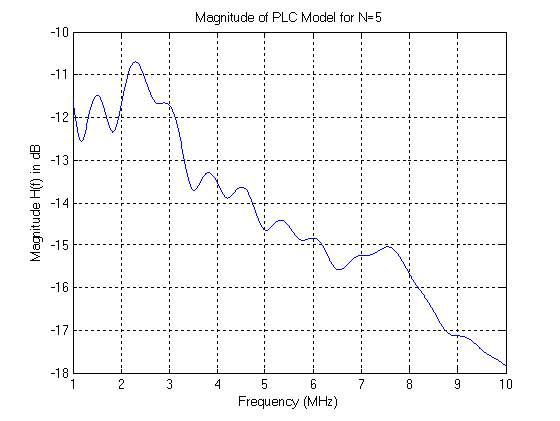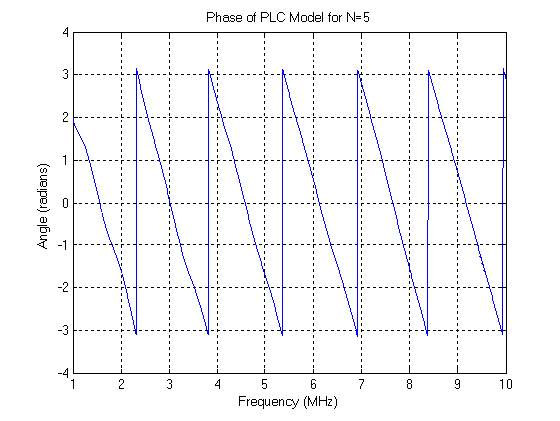Figure 5 and figure 6 shows the magnitude response and phase response of the PLC mathematical model respectively. The Magnitude response is done for 1MHz to 50MHz, and it shows how the magnitude of a signal behaves with respect to frequency when it is transmitted on a PLC channel for the derived frequency function H(f). For this case, the magnitude response does not display notches.  Phase response shows how phase of a PLC signal behaves when transmitted on a channel with respect to frequency. For this, the phase response is measured in radians and the phase response of a signal is plotted with linear values of frequency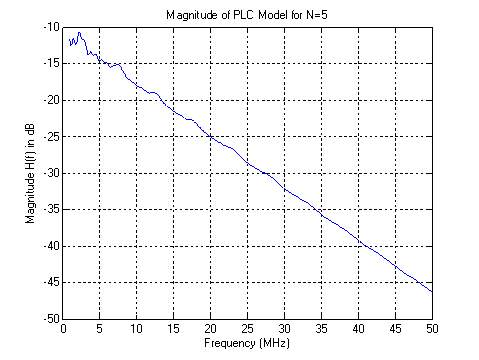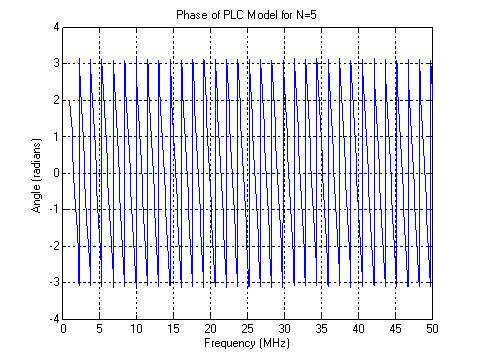### The Magnitude Response and Phase Response, PLC model for N=15

The following are the results for theoretical modelling for PLC model in the case when N=15. Figure 7 and figure 8 shows the magnitude response and phase response of the PLC mathematical model respectively. The Magnitude response is done for 1MHz to 50MHz frequency range, and it shows how the magnitude of a signal behaves with respect to frequency when it is transmitted on a PLC channel for the derived frequency function H(f) in dB. For this case, the magnitude response does display notches for 5 MHz, 10 MHz, 18-22 MHz and 30-35 MHz Phase response shows how phase of a PLC signal behaves when transmitted on a channel with respect to frequency. For this case, the phase response is measured in radians and the phase response of a signal is plotted with linear frequency range.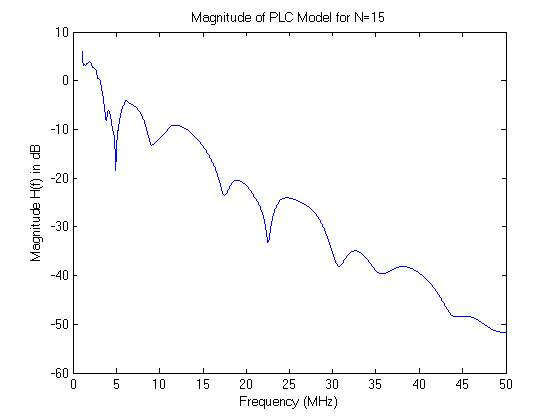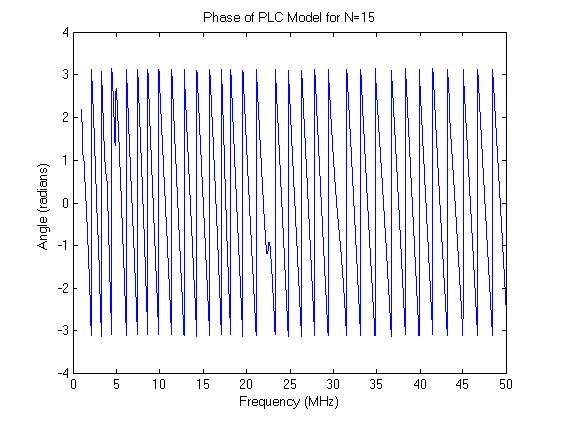### PLC Channel Simulink Model Simulations

The injected signal is injected in phase A of high voltage power network between Distributed lines and high voltage side of 3 phase transformer 2 n.ext to generation section and it is received on the same phase A between branch line 1 and high voltage side of the 3 phase transformer 1 using a capacitive coupler to enable injection and receiving communication signals on both the transmitting and receiving ends as shown in figure below.

The same harmonic signal can too be injected at any phase or lines like the phase B and C. The received signal is analysed using spectrum analyzer. The distribution line ranges from 50 Km to 10 Km where 50 Km line is the main high voltage (HV) transmission line.  The voltage levels and conductors’ type of selected HV power network consists of 220kVL-L, via a 0.415kV/220kV step up transformer, based on electrical power transmission standards.  In this proposed PLC channel model, the distribution line distance is 50km and it is terminated at a 40kW load with number of branches or bridge taps coming from the 50 km distribution line.

The HV access line is characterized by distributed parameter line (DPL).  The parametric values of the transmission line and the 6 branches are given below;

• Branch 1 has 25kW active power, 38 inductive reactive power, and 95 capacitive reactive power load
• Branch 2 has 30kW active power, 40 inductive reactive power, and 98 capacitive reactive power load
• Branch 3 has 20kW active power, 35 inductive reactive power, and 45 capacitive reactive power load
• Branch 4 has 50kW active power, 75 inductive reactive power, and 100 capacitive reactive power load
• Branch 5 has 60kW active power, 85 inductive reactive power, and 100 capacitive reactive power load
• Branch 6 has 38kW active power, 42 inductive reactive power, and 98 capacitive reactive power load

A3-phase, 60Hz step up and step down transformers are used in the Simulink PLC model where both are two-winding transformers and have equal values of 0.20 p.u resistance and 0.008p.u inductance. The transformer magnetizing resistance is equal to 500 p.u and 0.5 p.u magnetizing inductance. Transformer 2 is supplied by Y connected 3-phase 0.415kV source and transformer 1is a step down and it is supplied by 220kV to step it down to 0.415kV.

The model parameters of sending and receiving end capacitive coupling devices include; for the RL parallel branch parameters, R=1kΩ, L-10mH and for LC series branch, L=10mH, 33nF. The isolation transformer has parameters like; turn ration 1:1, magnetizing resistance is 500p.u and inductance of 0.5p.u.

The injected harmonic signal is done for an amplitude value of 1V to the parallel RL branch followed by coupling transformer and then the output is channeled to the distributed line through a combination of series/parallel branches circuit via an RL branch 5 shown in the figure below. Both coupling transformers device are grounded with1.6kΩ resistance.  A 60Hz filter is introduced at the output of the coupling transformer. In the PLC modelling, the transceiver coupling interface used at the receiving end has similar value as that of capacitive coupling transmitting side. The transfer function plot of capacitive coupling device and High Voltage network (through injecting signals via capacitive coupling device) is illustrated and shown in figure 9 below.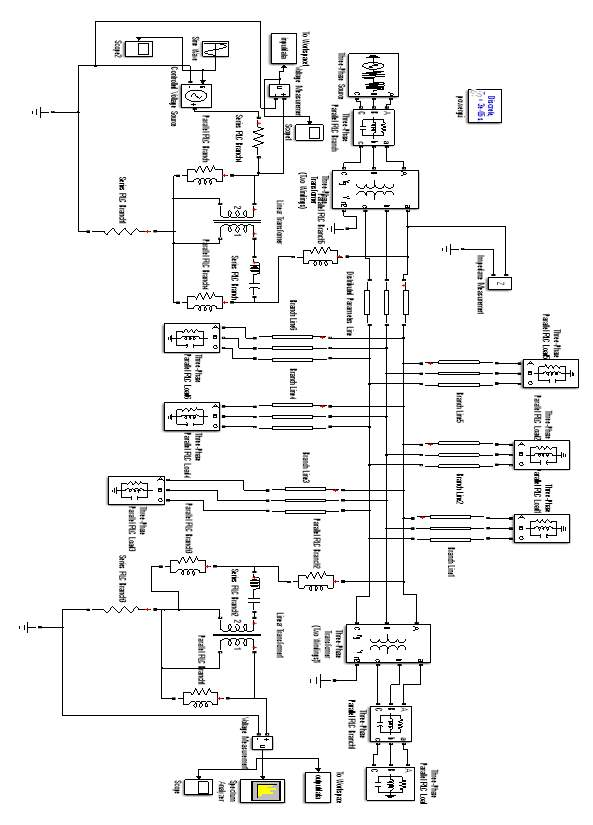### Simulation Results without Harmonics

Modelling of PLC channel using Transmission line theory discussed in the previous section of Mathematical modelling of a Power Line Communication (PLC), the graph shown in figure 10 below indicating the transfer function plot of the channel without injection of order harmonics. It shows the attenuation in the channel transfer function plots caused by constant impedances. In comparison with theoretical transfer function’s plot, the simulated transfers function if much close and accurate to the theoretical value and plots thus validating the proposed procedure and techniques for the characterization of modeling PLC system in power transmission lines.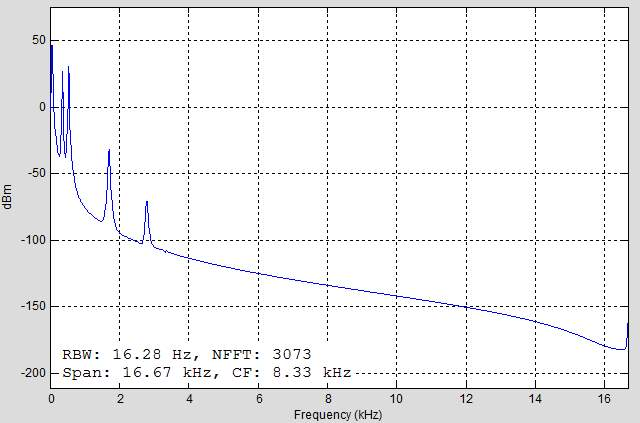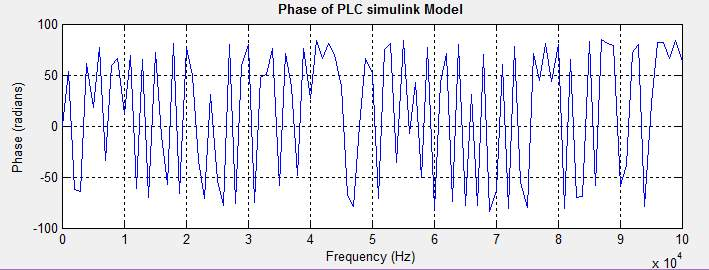In comparison, the phase response for the PLC Simulink model is similar with that plotted for the theoretical values and modelling. This is due to the fact that the modeled PLC Simulink involve an interconnected power lines and therefore the transfer function determined shows that is possible to model an existing power line for PLC lines.

Our academic experts are ready and waiting to assist with any writing project you may have. From simple essay plans, through to full dissertations, you can guarantee we have a service perfectly matched to your needs.

### Simulation Results with introduction of Harmonics

#### Simulation for Low Order Harmonics

With injection of 3rd, order harmonics, the gain of the transfer function gets attenuated deeply with high end notches. The degree of attenuation after the injection of harmonics into the power line communication is high than the attenuation when just incorporating impedances and loading variations. The plots showed in figure below indicate deep nulls or notches at frequencies for 1kHz- 6kHz ranges.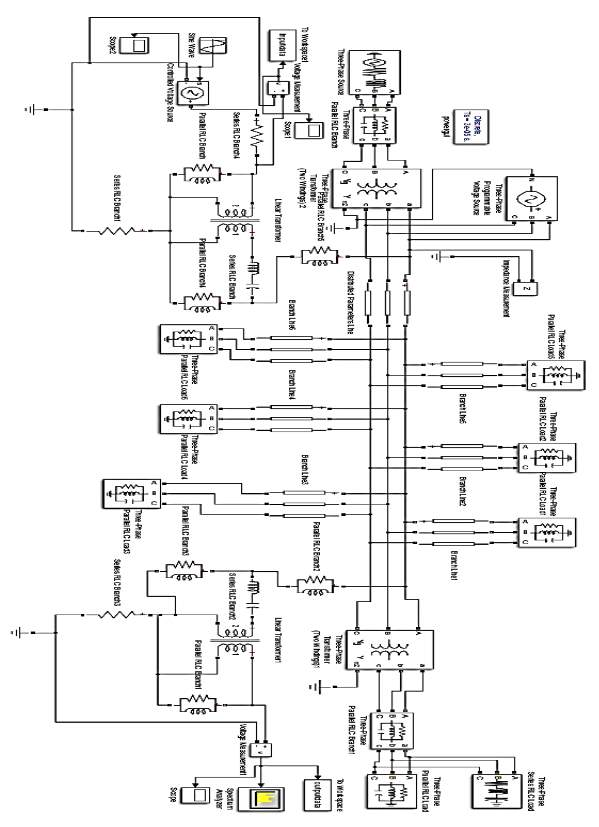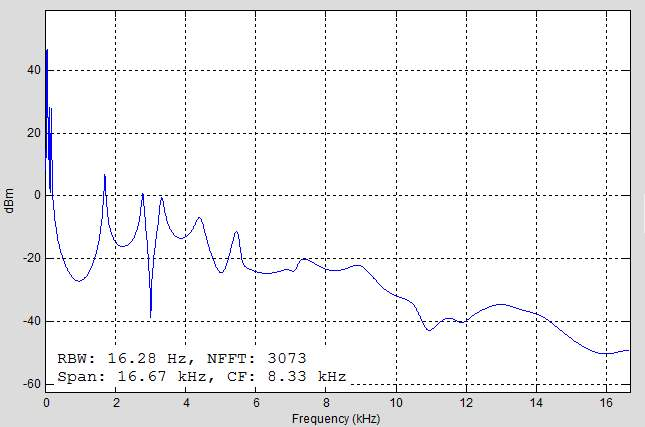#### Simulation for High Order Harmonics

With injection of high order harmonics (9th and 7th order harmonics), the gain of the transfer function gets attenuated deeply with high end notches that that of low order harmonic simulations. In high order harmonic simulation, the degree of attenuation during the injection of harmonics into the power line communication are still high than the attenuation when just incorporating impedances and loading variations or with low order harmonics. The plots showed in figure below indicate deep nulls or notches at frequencies for 1kHz- 8kHz frequency range and with shallow notches for high frequencies above 10kHz.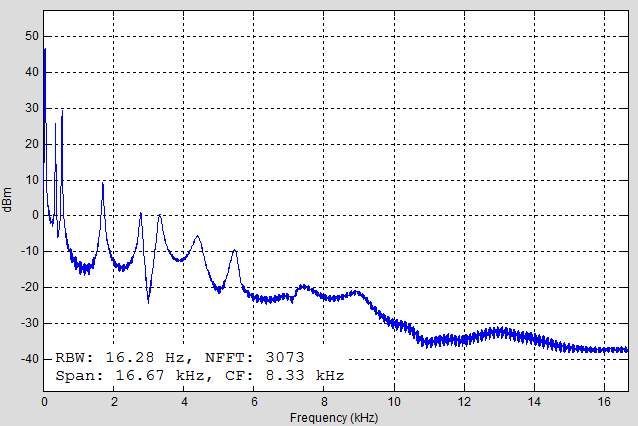For all cases of low order and high order harmonics are obtained from the Simulink PLC model and the frequency selective impedance for the case of transmission line theory are in good and agreeable therefore it validates the proposed modelling technique for PLC channel implementation in real power lines and power access networks for communications.

## Future work & Research

Michael Pecht (2019)  says that “following are the state-of-the-art advances in power line communication and its applications and outline the possible future research directions.

Channel measurement, modeling and interoperability (EMC), Advanced PLC technologies (PHY, MAC and networking including routing, QoS, QoE, security and privacy)

PLC networks (heterogeneous networks including PLC-VLC; and PLC-radio, interoperability, cognitive approaches)

PLC for smart grids, smart metering and grid control

Other PLC applications (IoT, Vehicular, etc.)

Open challenges, results and the role of PLC in new standards”

## Conclusion

With the extensive advancement in power lines interconnections and networks developments, it is therefore reliable and stable way to model a communication system and utilize the available power lines as a medium for communication signal transmission. This paper focused on the PLC modelling for high voltage access network and analysis of the impacts of high frequency harmonics. The PLC channel transfer function results are obtained by utilizing the transmission line theory and a PLC Simulink model. A capacitive coupling device is designed to inject a PLC signal into the high voltage PLC power network, and it was determined that the mathematical modelling for the PLC channel theoretical plots are approximately similar to that of Simulink PLC model but less attenuation were observed in the theoretical plots. With injection of frequency harmonics, the communication signal will be attenuated by half value. My proposed PLC Simulink model, it can be deduced that the any High voltage (HV) communication can be modeled by varying the parameters of the access network.

## Appendices

Simulation code for the PLC channel model and case of N=5

clear all;

clc;

%%%%%%_PLC simulations for multipath channel_%%%%%%

N=5;            %number of paths

a0=0;           %attenuation parameters

a1=1.65e-9;     %attenuation parameter

k=1;

%%%%path parameters%%%%%%

g(1:N)=[0.09,-0.012,0.012,-0.012,0.022];

d(1:N)=[100,130,160,190,300];

freq=1:0.01:50;           %frequency range

f=100:5000;

for m=1:N

H(f,m)=g(m).*exp(-(a0+a1.*((freq.*1e6).^k)).*d(m)).*exp(-2i.*pi.*(freq.*1e6).*(d(m)./vp));

end

H0(f)=H(f,1)+H(f,2)+H(f,3)+H(f,4)+H(f,5);

MagH(f)=10*log10(abs(H0(f)));

angH(f)=angle(H(f,1)+H(f,2)+H(f,3)+H(f,4)+H(f,5));

plot(freq,MagH(f))

title(‘Magnitude of PLC Model for N=5’);

xlabel(‘Frequency (MHz)’);

ylabel(‘Magnitude H(f) in dB’);

grid on

hold on

figure

plot(freq,angH(f))

title(‘Phase of PLC Model for N=5’);

xlabel(‘Frequency (MHz)’);

Simulation code for the PLC channel model and case of N=15

N=15;

a0=0;

a1=2.5e-9;

k=1;

vp=1.53e8;

g(1:N)=[1.832,0.516,0.765,-1.031,-0.8,-0.711,0.676,-0.676,1.263,-0.622,1.156,-.0978,0.747,-1.049,0.871];

d(1:N)=[113.2,90.1,101.8,143,148,200,261,322,411,490,567,740,960,1130,1250];

freq=1:0.01:50;

f=100:5000;

for m=1:N

H(f,m)=g(m).*exp(-(a0+a1.*((freq.*1e6).^k)).*d(m)).*exp(-2i.*pi.*(freq.*1e6).*(d(m)./vp));

end

H_F(f)=H(f,1)+H(f,2)+H(f,3)+H(f,4)+H(f,5)+H(f,6)+H(f,7)+H(f,8)+H(f,9)+H(f,10)+H(f,11)+H(f,12)+H(f,13)+H(f,14)+H(f,15);

MagH(f)=10*log10((H_F(f)));

angH(f)=angle(H_F(f));

figure

plot(freq,MagH(f))

title(‘Magnitude of PLC Model for N=15’);

xlabel(‘Frequency (MHz)’);

ylabel(‘Magnitude H(f) in dB’);

hold on

figure

plot(freq,angH(f))

title(‘Phase of PLC Model for N=15’);

xlabel(‘Frequency (MHz)’);

## Related Services

View all

### DMCA / Removal Request

If you are the original writer of this essay and no longer wish to have the essay published on the UK Essays website then please:

Related Services